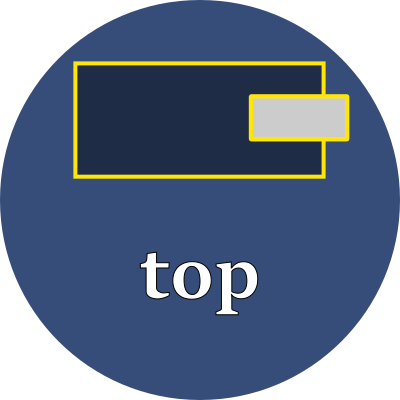# topExplicitly set the top edge of the element.

## Property values

This property has different interpretations, depending upon the element's `position` property.

position interpretation
static This property is ignored.
relative Shifts the normal flow-of-text position by the given amount. Positive values shift it down from the top. Negative values shift it outside the element's defined top boundary.
fixed Sets the top edge of the element to be the given amount above the top edge of the browser's viewport.
absolute Sets the top edge of the element to be the given amount above the top edge of the ancestor's viewport, but only if that ancestor has a position that is `relative`, `fixed` or `absolute`.
sticky Follows the rules for `relative` until the top value is scrolled to. After that, follows the rules for `fixed` and uses the given value as a fixed amount from the browser's top edge.

### Units

Use any of these font-relative typographic units to set the top:

 em em The `font-size` of the current font rem relative em The `font-size` of the element lh line height The `line-height` of the current font rlh relative line height The `line-height` of the element cap cap height The nominal height of the current font's capital letters ex "x" The height of the current font's "x" glyph

Or use any of these fixed size units which are independent of the current font:

 % percent A percentage of the parent element's height in inch A size equal 96px px pixels A size equal to 1/96 inch pt point A size equal 1in/72 = 1.33px pc pica A size equal 1in/6 = 12pt = 16px cm centimeter A size equal to 96px/2.54 = 37.8px mm millimeter A size equal to 96px/25.4 = 3.8px Q quarter millimeter A size equal to 96px/1016 = 0.94px

## Examples

style > position > topTop edge of element